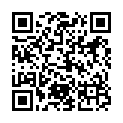# North Coast Synthesis Ltd.

## A♭+2+11+♯2Choose audio sample set:
MSK 007 Leapfrog VCF (patch; see in online store)
MSK 009 Coiler VCF (patch; see in online store)

Equivalent chord symbols: A♭+2+4+♯2, A♭+4+9+♯2, G♯+2+4+♯2, G♯+4+9+♯2, G♯+2+11+♯2.

Notes in this chord: A♭, B♭, B, C, E♭, D♭. Integer notation: {0, 1, 3, 8, 10, 11}.

Nearby chords (one less note): A♭+2+4, A♭+2+♯2, A♭+4+♯2, A♭m+2+4, A♭4+2+♯2, B♭m4+2+♯1.

Nearby chords (one more note): A♭11+♯2, A♭M11+♯2, B♭m11+♯1, G♭13-1+♯4, A♭+2+4+♯1+♯2, A♭+2+4+♯2+♯4.

Parallel chords (same structure, different root): C+2+11+♯2, D+2+11+♯2, E+2+11+♯2, F+2+11+♯2, G+2+11+♯2, A+2+11+♯2, B+2+11+♯2, C♭+2+11+♯2, D♭+2+11+♯2, E♭+2+11+♯2, F♭+2+11+♯2, G♭+2+11+♯2, B♭+2+11+♯2, C♯+2+11+♯2, D♯+2+11+♯2, E♯+2+11+♯2, F♯+2+11+♯2, G♯+2+11+♯2, A♯+2+11+♯2, B♯+2+11+♯2.

Experimental fretting charts for guitar standard EADGBE tuning (change tuning or instrument):